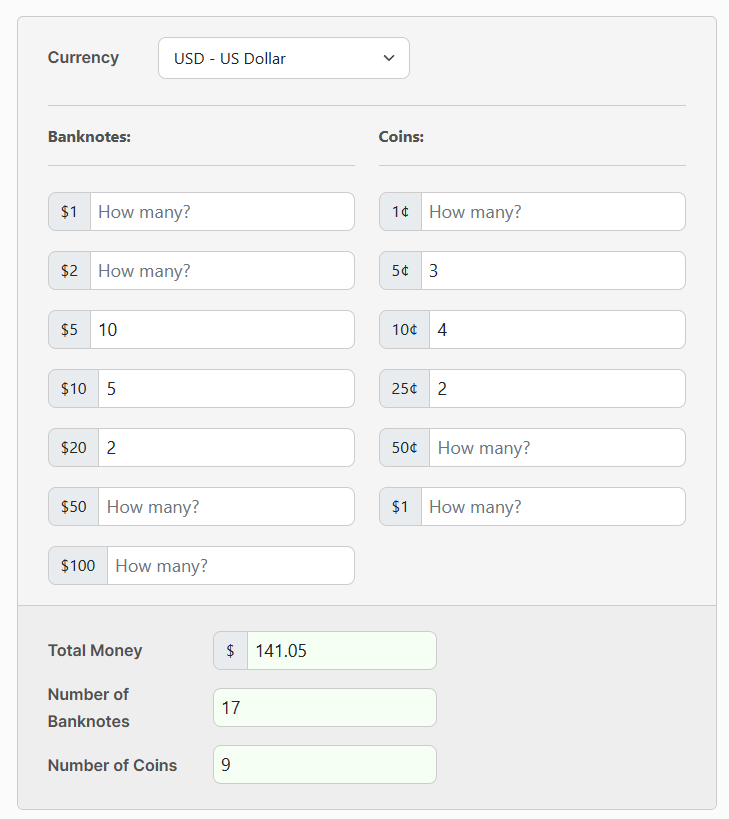# Money Calculator

Specify the number of banknotes and coins to easily calculate the total amount of cash.

Banknotes:
\$1
\$2
\$5
\$10
\$20
\$50
\$100
Coins:
10¢
25¢
50¢
\$1
\$

The Money Calculator allows you to calculate the total amount of money based on the entered quantity of banknotes and coins of a given type. In addition, the calculator also counts the total number of banknotes and coins.

## How to use the Money Calculator?

To use this calculator:

1. Select the currency (e.g. US Dollar).
2. Enter the selected quantity of banknotes and coins of a given type.
3. Check the result in the "Total Money" field.

For example:## How to calculate the total amount of money?

1. Gather all bills and coins.
2. Separate bills and coins by value.
3. Count the number of bills for each value and multiply by the value (e.g. 10 x \$20 = \$200 for ten \$20 bills).
4. Add up the total value of the bills.
5. Count the number of coins for each type and multiply by the value (e.g. 25 x \$0.25 = \$6.25 for twenty-five quarters).
6. Add up the total value of coins, then add to the total value of bills for the overall total.

Alternatively, you can use a money calculator for an automated total count of your bills and coins.

Financial Calculators
PureTables.com
Have an idea for a tool or calculator that would make your life easier? Contact us, so we could try to create and provide it.